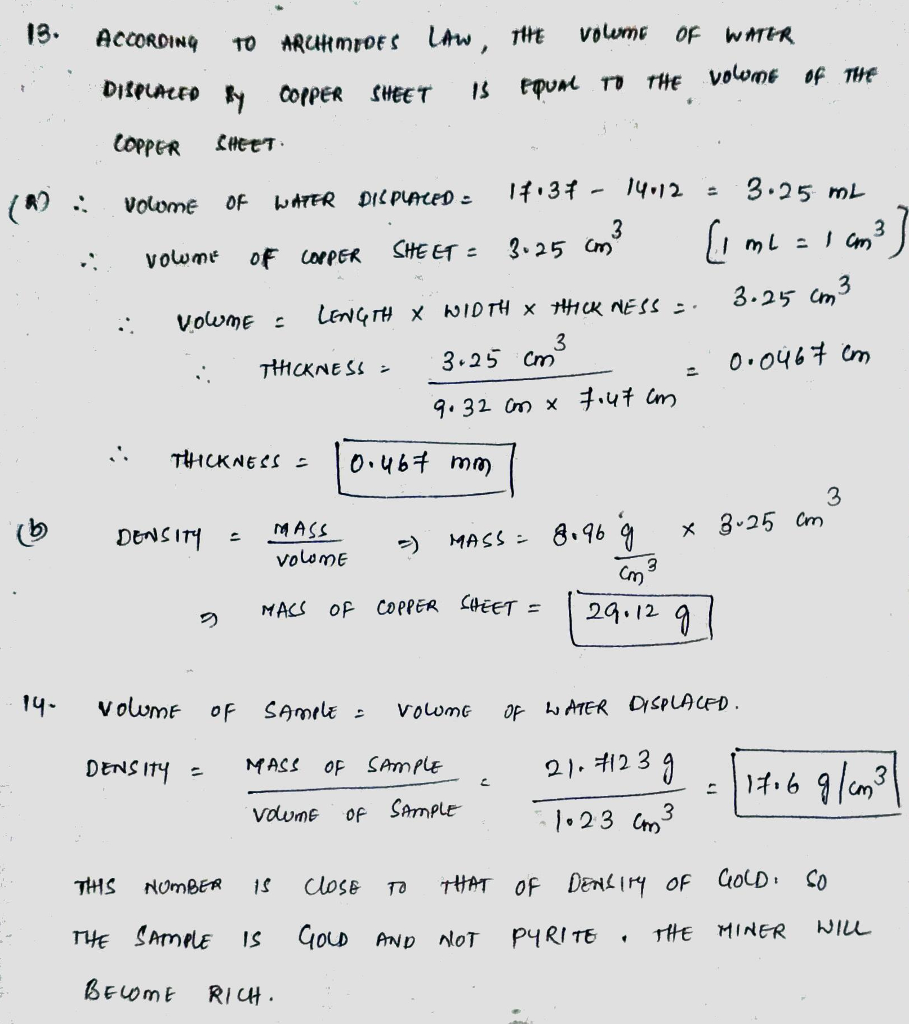# Homework Solution: You find the length and width of a rectangular sheet of copper are 9.32 cm and 7.47…

You find the length and width of a rectangular sheet of copper are 9.32 cm and 7.47 cm, respectively. You are unable to directly measure the third side, so you decide to find out how much water the sheet displaces. Without the sheet in the graduated cylinder, the volume of water is 14.12 mL. With the sheet in the cylinder, the volume of water is now 17.37 mL. a. What is the thickness of the sheet (in mm)? b. If the density of copper is 8.96 g/cm^3, what is the mass of the rectangular sheet? A miner discovers a large quantity of gold-colored rocks in a ravine, but does not know whether it is pyrite ("fool's gold") or gold. He drops a sample into a graduated cylinder and discovers that it displaces 1.23 mL of water. If the sample has a mass of 21.7123 g, will the miner become rich or stay poor? Justify, if you know that pyrite has a density of 5.01 g/cm^3, and gold has a density of 19.3 g/cm^3.